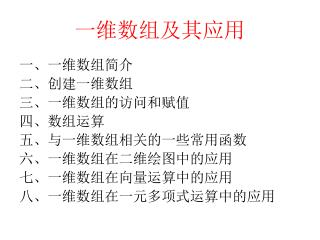DownloadDownload Presentation# 一维数组及其应用

Télécharger la présentation## 一维数组及其应用

- - - - - - - - - - - - - - - - - - - - - - - - - - - E N D - - - - - - - - - - - - - - - - - - - - - - - - - - -
##### Presentation Transcript

1. 一维数组及其应用 一、一维数组简介 二、创建一维数组 三、一维数组的访问和赋值 四、数组运算 五、与一维数组相关的一些常用函数 六、一维数组在二维绘图中的应用 七、一维数组在向量运算中的应用 八、一维数组在一元多项式运算中的应用

2. n个元素排成一行，又称为行向量（row vector） 常被 看作为 1 X n 矩阵（二维数组） 一、一维数组简介 1、行数组

3. n个元素排成一列，又称为列向量(column vector)，常看作为 n X1 矩阵（二维数组） 　　在Matlab中提到向量，一般都是指的列向量 2、列数组

4. 3、标量（scalar） 一个数，称为标量，在Matlab中看作为 1 X 1 矩阵（二维数组） a = 在matlab中，使用a(1)和a(1,1)都可以访问变量a Matlab中，标量和向量一般用小写字母表示

5. 二、创建一维数值数组 1、用方括号[ ]创建一维数组 • 整个数组放在方括号里 • 行数组元素用空格或逗号分隔 x = [ 2, pi/2, sqrt(3), 3+5i ] • 列数组元素用分号分隔，分号起换行 y = [ 2; pi; 3/4; j ] • 标点符号一定要在英文状态下输入

6. 2、用[ ]由小数组生成大数组 （1）执行如下赋值语句： （3）再执行如下赋值语句： a = [ 1, 3 ] b = [ 2, 4 ] c = [ 3; 5 ] g = [ c; c ] h = [ g; g ] （2）然后执行如下赋值语句： 此时： g = ? h = ? d = [ a, a ] f = [ d,b ] 此时：d = ? f = ?

7. 3、用[ ]生成空数组 x = [ ] 　　方括号内无字符，或只包含空格，则生成空数组。此时在matlab内部生成一个double类型的变量x，其内容为空，占用0字节。空数组在进行数组并置操作（二个小数组合并为一个大数组）时，经常用到。 （1）执行如下赋值语句： （2）然后执行如下赋值语句： x = [ ] a = [ 2, 4 ] b = [ 3,5 ] x = [ x, a ] x = [ x, b ] 此时：x = ?

8. 4、用冒号:创建一维数组 x = a:b x = a:inc:b a，b必须为实数,若未提供inc的数值，则inc = 1 a是数组x中的第一个元素，b不一定是数组x的最后一个元素。 　　若inc取的不合适，可能会生成空数组。 生成如下数组 x = [a, a+inc, a+2*inc, … ] x = 1:10 y = 10:-2:-10 z = [ 1:2:99, 2:2:100 ] w = 2:3:-10 x = ? y = ? z = ? w = ?

9. 5、用linspace()函数创建一维数组 x = linspace(a,b) a，b可以为复数 x = linspace(a,b,n) 　　在a和b之间，均匀生成 n (未提供n时，n=100)个数据，包括a和b在内。 若a，b为实数，等效于： 执行如下赋值语句后，x = ? y = ? x = linspace(0, 8, 17) y = linspace(1+i, 4+4*i, 7)

10. 6、用logspace()函数创建一维数组 x = logspace(a,b) x = logspace(a,b,n) 　　先在a和b之间均匀生成n个数据，包括a和b，然后以这n个数据为指数，生成一维数组x。 例：x = logspace(1,2,6)生成的数组为： x=[101, 101.2, 101.4, 101.6, 101.8, 102 ] =[10.0000, 15.8489, 25.1189, 39.8107, 63.0957, 100.0000] x = logspace(a,pi,n)

11. 三、一维数组的访问和赋值 b = x(end) end是数组x的最后一个元素的下标 引用数组 x 中的最后一个元素，将其赋值给变量 b 1、引用一维数组中的单个元素 x = 1:5 k = 2 a = x(k) 引用数组 x 中的第 k 个元素，将其赋值给变量 a Matlab中数组元素的下标从 1 开始 c = x(8)会出现什么结果？

12. 2、一维数组中单个元素的赋值 x = 1:5 a = 1.5 k = 3 x(k) = 0 将 0 赋值给数组 x 中的第 k 个元素 x(end) = a 将 a 赋值给数组 x 中的最后一个元素 x(8) = 6.5 会出现什么结果？

13. 3、引用一维数组中的子数组 引用一维数组x中的第2、5、9个元素，将其赋值给变量 a x = linspace(1,5) s = [2,5,9] a = x(s) x = linspace(1,5) a = x([2,5,9]) b = x(end:-1:1) c = x(1:2:end) d = x(2:2:end) b = ? c = ? d = ?

14. 4、一维数组中子数组的赋值 x = linspace(1,5) s = [1,4] a = [2,7] x(s) = a x = linspace(1,5) x([1,4]) = [2,7] 将2、7分别赋值给x的第1、4个元素

15. 5、利用空数组进行赋值可以修改数组的大小 （1）执行如下赋值语句： x = 10:10:70 x(2) = [ ] 此时：x = ? （2）再执行如下赋值语句： x([2,4]) = [ ] 此时：x = ?

16. 四、数组运算 数组运算是Matlab软件定义的运算规则，其目的是为了数据管理方便、操作简单、指令形式自然和执行计算的有效。 无论在数组上施加什么运算(加减乘除或函数)，总认定那种运算对被运算数组中的每个元素(Element)进行运算。

17. 1、一维数组的转置运算 x.’ x’ 对x做转置（行→列，列→行） 对x做共轭转置 若x的元素均为实数，则x.’与x’的结果相同 y = x.’ 对数组x做转置运算，将运算结果赋值给变量y，内存中数组x的内容并没有任何变化。 x1 = (1:10).’ y1 = (1:10)’ x2 = linspace(i,10*i).’ y2 = linspace(i,10*i)’

18. 2、一维数组的数学运算 (1) 两个一维数组之间的数学运算 • x + y：加，对应位置的数组元素相加 • x - y：减，对应位置的数组元素相减 • x.*y：点乘，对应位置的数组元素相乘 • x./y：右点除，对应位置的数组元素相除 • x.\y：左点除 • x.^y：点幂，对应位置的数组元素做幂运算 一维数组x、y 维数相同 时，可以进行如下如下的数组运算： 运算结果为与x、y同维的数组

19. (2)一维数组与标量 之间的数学运算 x+c ：x的每个元素加c x-c： x的每个元素减c x.*c 或 x*c：x的每个元素乘c， x./c 或 x/c： x的每个元素除以c x.\c 或 x\c：c除以x的每个元素 x.^c：点幂， x的每个元素做幂运算 c.^x：点幂，c做幂运算 x为一维数组，c为一个数(标量)，x和c之间可以进行： 运算结果为与x同维的数组

20. 练习：试写出下列matlab语句的输出结果，并在matlab中进行验证。练习：试写出下列matlab语句的输出结果，并在matlab中进行验证。 a = [1,1, 1] b = [1, 0, 0] c = [ 0, 0, 0] d = (a+b).*(a-b) e = (d + 1) + (d - 1)*i f = e.’./e’ + e.’.\e’ g = b.^d + d.^b

21. 3、一维数组的关系运算 (1)两个一维数组之间的关系运算 进行比较的两个数组必须维数相同，比较在两数组相同位置上的元素间进行，返回一个由0和1组成的“逻辑数组” 。

22. (2)一维数组和标量之间的关系运算 标量可以与任何维数组进行比较。比较在此标量与数组每个元素之间进行，因此比较结果与被比数组同维，返回一个由0和1组成的“逻辑数组” 。

23. (3)关系运算中的注意事项 比较两个数是否相等的关系运算符是“ == ”，单个的等号“ = ” 是变量赋值符号。 不等于的关系运算符是“ ~= ”，不要与 C 语言的不等于符号“ != ” 混淆。 比较两个浮点数是否相等时需要注意，由于浮点数的存储时的误差的存在，在程序设计中要避免直接比较两个浮点数是否相等，而是采用大于、小于的比较运算将待确定值限制在一个满足需要的区间之内。 3<x<7这样的数学表达式必须用逻辑“与”运算才能正确表示。

24. 4、一维数组的逻辑运算 逻辑运算可以在标量与标量，数组与数组，标量与数组间进行 a = [0, 1, 2, 0, 3], b = [4, 5, 0, 0, 6], c = 7 在逻辑表达式中，作为输入的任何非 0 实数都被看作是“逻辑真”，只有 0 才被认为是“逻辑假”。 　　标量（一个数）可以与任何维数组进行逻辑运算。运算在标量与数组每个元素间进行。 　　当两个数组进行逻辑运算时，参与运算的数组必须维数相同，运算在两数组相同位置上的元素间进行。

25. 5、逻辑数组用作 “逻辑索引” A = 1:10 L = logical([0 1 0 1]) A(L) 逻辑索引：通过逻辑数组 L 中“逻辑1”所在的位置，指出数组 A 中元素的的位置，从而可以对A 数组中的元素（子数组）进行访问或赋值。 L 的元素个数应等于或小于 A 的元素个数，一般情况下，L 应是与 A 同维的逻辑数组

26. 6、函数作用在数组上的运算规则 Matlab中的很多函数都可以直接以数组作为输入参数，函数会作用在数组的每个元素上。 输入为行数组，则输出也为行数组 输入为列数组，则输出也为列数组

27. 关系、逻辑运算举例 　　在100, 103, 106, 109, ... , 148, 151这些整数中，能被 7 整除的数有哪些？ A = 100:3:151 B = rem(A,7) L = B==0 r = A(L) 100以内的哪些正整数满足：0.7 < sin(n) < 0.75 ？ n = 1:100 L1 = sin(n)>0.7 L2 = sin(n)<0.75 L = L1&L2 r = n(L)

28. 五、与一维数组相关的一些常用函数 当函数的输入参数为一维数组x时（x为行或列）：

29. 六、一维数组在二维绘图中的应用 x，y为同维的一维数组，二维绘图函数plot(x,y)的绘图原理：分别以x，y对应位置的元素为横坐标和纵坐标，得到n个数据点，描点，然后依次将第1,2,…,n点连线，绘制出二维图形。

30. x = 0:0.1:10*pi y = sin(2*x).*cos(x/2) plot(x,y) 数组乘法

31. 七、一维数组在向量运算中的应用

32. 1、向量的模（长度） a = [4,3,1] d = sqrt(sum(a.*a)) 或： d = sqrt(sum(a.^2))

33. 2、两点之间的距离 a = [4,3,1] b = [5,2,3] AB = sqrt(sum((a - b).^2))

34. 3、向量的方向角与方向余弦，单位向量 a = [4,3,1] d = sqrt(sum(a.^2)) e0 = a./d

35. 4、向量的线性运算 直接利用matlab的数组运算规则即可进行运算 a = [4,3,1] b = [5,2,3] c = 1.6 p = a + b s = a – b d = c*a

36. 5、两向量的数量积（内积/点积/点乘） Matlab中提供了 dot()函数实现两向量的数量积 = dot(a,b) = sqrt(dot(a,a))

37. 6、两个向量之间的夹角 tmp = dot(a,b)/sqrt(dot(a,a))/sqrt(dot(b,b)) theta = acos(tmp)

38. 7、两向量的向量积（叉乘） Matlab中提供了 cross()函数实现两向量的向量积 = cross(a,b)

39. 8、向量的混合积： = dot(cross(a,b),c)

40. 上机练习：

41. 3.以A(1,2,3)，B(2,0,5)，C(4,2,-1)为顶点的三角形的面积。3.以A(1,2,3)，B(2,0,5)，C(4,2,-1)为顶点的三角形的面积。 4.求以A(0,0,2)，B(3,0,5)，C(1,1,0)，D(4,1,2)为顶点的四面体的体积。

42. 八、一维数组在一元多项式运算中的应用 　　借助matlab提供的函数，处理多项式是一件非常简单的事情，很容易对多项式进行积分、微分以及求根的操作。 一元多项式在代数中占有非常重要的地位。在实际应用中如对实验数据的插值、微商和曲线拟合等，都要大量用到多项式；在矩阵分析时，也要用到一元多项式的概念。多项式函数是形式最简单的函数，也是最容易计算的函数，从理论上讲，它可以表示绝大多数复杂函数。在许多计算机的计算和编程中，很多函数值如sin(x),cos(x)等的计算都是先将函数进行Tailor展开为多项式进行逼近计算的，并且都能达到很高的精度。

43. 在matlab中，一个一元多项式用一个行向量来表示的，向量元素为多项式系数的降幂排列，其中最后一个元素代表多项式中的0幂项。 在matlab中，一个一元多项式用一个行向量来表示的，向量元素为多项式系数的降幂排列，其中最后一个元素代表多项式中的0幂项。 1、多项式的表示和创建 p = [1,-12,0,25,116]

44. 2、多项式的符号表示 poly2sym() 把系数向量表示的多项式转为符号表示 poly2sym(p) 用默认字符’x’来表示多项式的变量 poly2sym(p,’v’) 用字符‘v’来表示多项式的便量 p = [1,-12,0,25,116] px = poly2sym(p) pt = poly2sym(p,’t’)

45. 3、计算多项式的值 p = [1,-12,0,25,116]; x1 = 2.3; x2 = [-1,3,7]; 计算多项式p在x处的值，x可以是标量，或数值数组 y = polyval(p,x) p1 = polyval(p,x1); p2 = polyval(p,x2);

46. 4、求多项式的根 r = roots(p) 此函数返回多项式p的根组成的向量 p = [1,-12,0,25,116] r = roots(p) 　　多项式和多项式的根都是用向量表示的，为加以区别，多项式的根表示成列向量。 　　对于系数为实数的多项式，如果其根出现复数，则复数必是成对出现的。